## 1. 图像卷积 - cv2.filter2D 函数

OpenCV 库，cv2.fliter2D 函数用于对图片根据特定的核(kernel) 进行卷积操作. 该函数可以将任意线性滤波器应用于图像. 支持 in-place 操作. 当卷积核超出图像时，该函数会根据指定的边界模式(border mode)插入特定的像素值.

cv.filter2D(src, dst, ddepth, kernel, anchor=(-1, -1), delta=0, borderType = BORDER_DEFAULT)
#ddepth – desired depth of the destination image;
#if it is negative, it will be the same as src.depth();
#the following combinations of src.depth() and ddepth are supported:
#src.depth() = CV_8U, ddepth = -1/CV_16S/CV_32F/CV_64F
#src.depth() = CV_16U/CV_16S, ddepth = -1/CV_32F/CV_64F
#src.depth() = CV_32F, ddepth = -1/CV_32F/CV_64F
#src.depth() = CV_64F, ddepth = -1/CV_64F
#when ddepth=-1, the output image will have the same depth as the source.

$$\texttt{dst} (x,y) = \sum _{ \stackrel{0\leq x' < \texttt{kernel.cols},}{0\leq y' < \texttt{kernel.rows}} } \texttt{kernel} (x',y')* \texttt{src} (x+x'- \texttt{anchor.x} ,y+y'- \texttt{anchor.y} )$$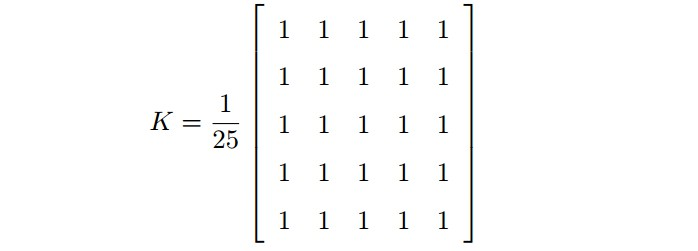import numpy as np
import cv2 as cv
from matplotlib import pyplot as plt

kernel = np.ones((5,5), np.float32)/25
dst = cv.filter2D(img, -1, kernel)
plt.subplot(121); plt.imshow(img); plt.title('Original')
plt.xticks([]); plt.yticks([])
plt.subplot(122); plt.imshow(dst); plt.title('Averaging')
plt.xticks([]); plt.yticks([])
plt.show()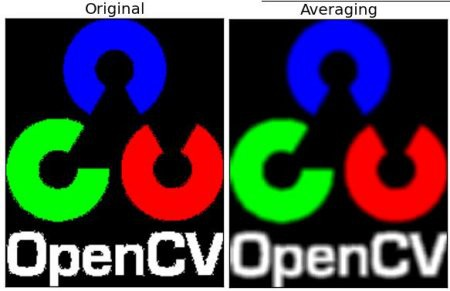## 2. 图像模糊 - 图像平滑

OpenCV 提供了四种图像模糊技术：

• 平均模糊
• 高斯模糊
• 中值模糊
• 双边滤波

### 2.1. 平均模糊

• cv2.blur(img, (5, 5)) - (5, 5) 是平滑内核大小，等同于 np.ones((5, 5), np.float32)/9.
• cv2.boxFilter() - 如果不使用归一化卷积框，则设置参数 normalize=False.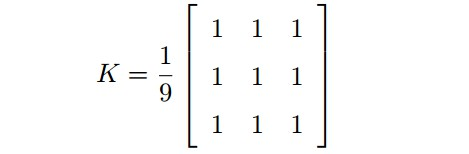import cv2
import numpy as np
from matplotlib import pyplot as plt

blur = cv2.blur(img,(5,5))

plt.subplot(121)
plt.imshow(img)
plt.title('Original')
plt.xticks([])
plt.yticks([])

plt.subplot(122)
plt.imshow(blur)
plt.title('Blurred')
plt.xticks([])
plt.yticks([])
plt.show()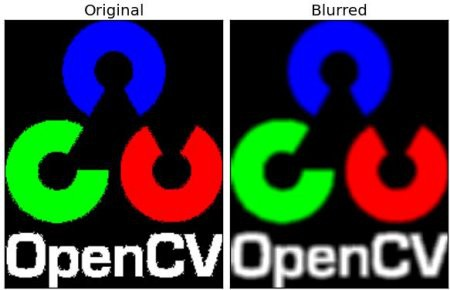### 2.2. 高斯模糊

cv2.GaussianBlur() 函数，需要指定高斯核的宽和高(必须是奇数)，以及高斯函数沿 X 和 Y 方向的标准差. 如果只指定了 X 方向的的标准差， Y 方向也会取相同值. 如果两个标准差都是 0，那么函数会根据核函数的大小自己计算.

#0 是指根据窗口大小(5,5)来计算高斯函数标准差
blur = cv2.GaussianBlur(img, (5,5), 0)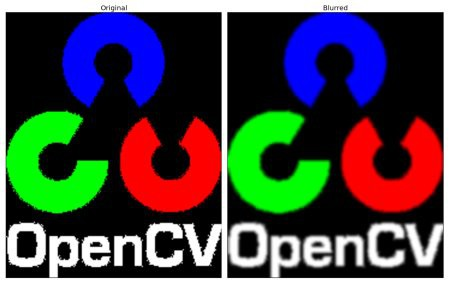### 2.3. 中值模糊

median = cv.medianBlur(img, 5)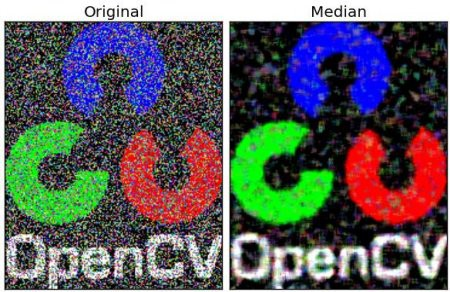### 2.4. 双边滤波

cv2.bilateralFilter() 能在保持边界清晰的情况下有效的去除噪音. 但是该滤波函数与其他滤波器相比会比较慢. 高斯滤波器是求中心点邻近区域像素的高斯加权平均值. 这种高斯滤波器只考虑像素之间的空间关系，而不会考虑像素值之间的关系(像素的相似度). 所以这种方法不会考虑一个像素是否位于边界. 因此边界也会别模糊掉，而这正不是想要的.

cv2.bilateralFilter(src, d, sigmaColor, sigmaSpace)

 - src - 源图像

 - d – Diameter of each pixel neighborhood that is used during filtering. 滤波器间使用的每个像素邻域的直径. (If it is non-positive, it is computed from sigmaSpace).

 - 最后两个 sigmaColor 参数 - 空间高斯函数标准差和灰度值相似性高斯函数标准差(如果它们很小(<10)，过滤器将不会有太多的效果，而如果它们很大(> 150)，将会产生非常强烈的效果，使得图像显得“卡通”.) 例如：

#9 邻域直径，两个 75 分别是空间高斯函数标准差，灰度值相似性高斯函数标准差
blur = cv2.bilateralFilter(img, 9, 75, 75)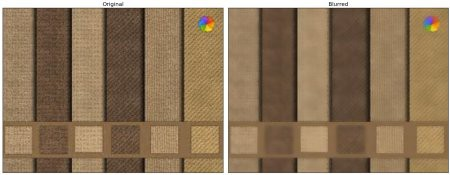import cv2
import numpy as np
from matplotlib import pyplot as plt

#blur = cv2.blur(img, (5, 5))
#blur = cv2.GaussianBlur(img, (5, 5), 0)
#median = cv2.medianBlur(img, 5)
blur = cv2.bilateralFilter(img, 9, 75, 75)

plt.subplot(121), plt.imshow(img)
plt.title('Original'), plt.xticks([]), plt.yticks([])
plt.subplot(122), plt.imshow(blur)
plt.title('Averaging'), plt.xticks([]), plt.yticks([])
plt.show()

## 3. 参考

 - OpenCV官方教程中文版(For Python) - 段力辉(译)

Last modification：May 27th, 2019 at 09:01 pm